# Jackson inequality

Jump to: navigation, search

An inequality estimating the rate of decrease of the best approximation error of a function by trigonometric or algebraic polynomials in dependence on its differentiability and finite-difference properties. Letbe a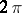-periodic continuous function on the real axis, let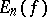be the best uniform approximation error ofby trigonometric polynomials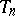of degree, i.e.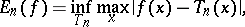and let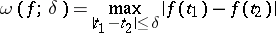be the modulus of continuity of(cf. Continuity, modulus of). It was shown by D. Jackson  that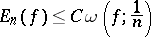(*)

(whereis an absolute constant), while ifhas an-th order continuous derivative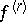,, thenwhere the constant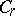depends ononly. S.N. Bernshtein  obtained inequality (*) in an independent manner for the caseIfis continuous ortimes continuously differentiable on a closed interval,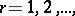and ifis the best uniform approximation error of the functiononby algebraic polynomials of degree, then, for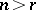one has the relation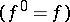where the constant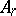depends ononly.

The Jackson inequalities are also known as the Jackson theorems or as direct theorems in the theory of approximation of functions. They may be generalized in various directions: to approximation using an integral metric, to approximation by entire functions of finite order, to an estimate concerning the approximation using a modulus of smoothness of order, or to a function of several variables. The exact values of the constants in Jackson's inequalities have been determined in several cases.